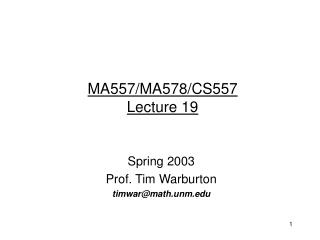Download PresentationMA557/MA578/CS557 Lecture 19

# MA557/MA578/CS557 Lecture 19

Download Presentation## MA557/MA578/CS557 Lecture 19

- - - - - - - - - - - - - - - - - - - - - - - - - - - E N D - - - - - - - - - - - - - - - - - - - - - - - - - - -
##### Presentation Transcript

1. MA557/MA578/CS557Lecture 19 Spring 2003 Prof. Tim Warburton timwar@math.unm.edu

3. DG Scheme for the Scalar ADE(Lax-Friedrichs flux – equivalent to upwind)

4. DG Derivative Operator • We are going to introduce a DG derivative operator to simplify the scheme definition. • The linear operator Dtilde is such that the following holds for all intervals Ij • With the choice of penalty terms tauL and tauR to be determined.

5. In Operator Notation Advectionterm(Lax-Friedrichs flux ~ upwind fluxfor scalar case) Diffusionterm

6. General Dtilde Operator • We previously defined Dtilde for the special case where the test function is compactly supported on the j’th cell. • We generalize by:

7. Skew-symmetry of Dtilde1,1 [integrate by parts]

8. Skew-symmetry • Note:the negative sign when the operator moves. • Trivially:

9. Recap • We already proved that the DG scheme, with Lax-Friedrichs fluxes, for advection (D=0) is stable in the sense that : • Adding the diffusion term (dropping boundary terms) gives: • In terms of norms:

10. Stability!! • Assuming D>=0 (reasonable since particles do not jump randomly with negative rates)

11. D operator • If we move to the Legendre basis we can write down a discrete description of Dtilde:

12. Implementation of Dtilde function [dfdx] = LEGdgderiv(f, nodex, tauL, tauR, D, F, G, H, J) [p,N] = size(f); % p = maximum polynomial order p=p-1; % N = number of nodes N=N+1; % space for derivative dfdx = zeros(p+1,N-1); % cells 2 to N-2 ids = (2:N-2); dfdx(:,ids) = (D*f(:,ids) + tauL*F*f(:,ids) + tauL*G*f(:,ids-1) + tauR*H*f(:,ids) + tauR*J*f(:,ids+1)); % cell 1 (periodicity assumed) dfdx(:,1) = (D*f(:,1) + tauL*F*f(:,1) + tauL*G*f(:,N-1) + tauR*H*f(:,1) + tauR*J*f(:,2)); % cell N-1 (periodicity assumed) dfdx(:,N-1) = (D*f(:,N-1) + tauL*F*f(:,N-1) + tauL*G*f(:,N-2) + tauR*H*f(:,N-1) + tauR*J*f(:,1)); % apply chain rule for physical cell width dx = nodex(2:N)-nodex(1:N-1); coeff = ones(p+1,1)*(2./dx); dfdx = dfdx.*coeff;

13. In Action (advection eqn) % TIME STEPPING tauL = (1/u)*(u+abs(u))/2; tauR = (1/u)*(u-abs(u))/2; for tstep = 1:Ntsteps sigma = rho; for rkstage = 3:-1:1 dsigmadx = LEGdgderiv(sigma, nodex, tauL, tauR, D, F, G, H, J); sigma = rho + (dt/rkstage)*(-u*dsigmadx); end rho = sigma; if ( ~mod(tstep, 100) ) q = V*rho; % plot(x(:),abs(q(:)-exp(-(x(:)-dt*tstep).^2))); plot(x,q); pause(0.1); tstep*dt end end

14. Discrete Scheme • Step 1: q = x DG derivative of C • Step 2: time rate of change of C Advectionterm(Lax-Friedrichs flux ~ upwind fluxfor scalar case) Diffusionterm

15. Dropping q • We can do away with q: • This should make the following observation clear. The dt dependence for stability is now: • The first term is due to the spectral radius of the advection operator. • The second term is due to the spectral radius of the diffusion operator.

16. In Action (ADE) % TIME STEPPING for tstep = 1:Ntsteps sigma = rho; for rkstage = p:-1:1 % advection: upwind bias on flux terms dsigmadx = LEGdgderiv(sigma, nodex, tauL, tauR, D, F, G, H, J); % diffusion: centered fluxes dsdx = LEGdgderiv(sigma, nodex, 1, 1, D, F, G, H, J); d2sdx2 = LEGdgderiv(dsdx, nodex, 1, 1, D, F, G, H, J); sigma = rho + (dt/rkstage)*(-u*dsigmadx+Dcoeff*d2sdx2); end rho = sigma; if ( ~mod(tstep, 400) ) q = V*rho; plot(x,q); axis([xmin xmax 0 1]) pause(0.1); end end